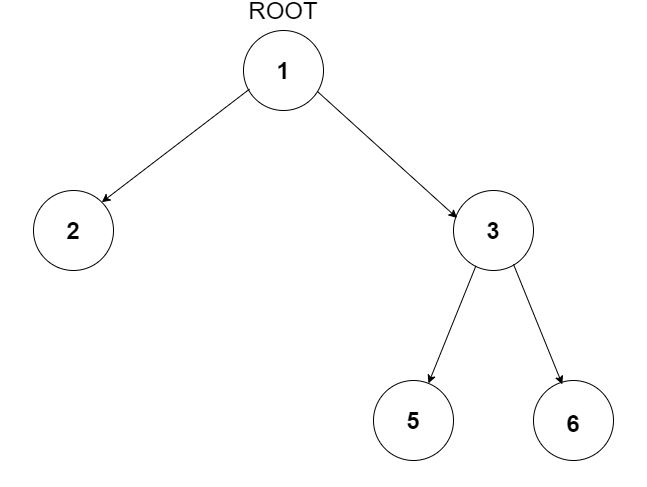Problem title
Difficulty
Avg time to solve

Point to Greatest Value Node
Easy
10 mins
Diagonal Traversal
Moderate
15 mins
Sub Query Sum
Easy
10 mins
Check if the Word is present in Sentence or not
Easy
15 mins
Create Sequence
Easy
10 mins
Closest Binary Search Tree Value
Easy
15 mins
Easy
10 mins
Exactly One Child
Easy
15 mins
Predecessor and Successor In BST
Moderate
25 mins
Pair sum in a BST
Easy
15 mins8

# Minimum Depth Of Binary Tree

Difficulty: MEDIUM
Avg. time to solve
20 min
Success Rate
80%

Problem Statement

#### You have been given a Binary Tree of integers, find the minimum depth of this Binary Tree. The minimum depth of a Binary Tree is the number of nodes along the shortest path from the root node down to the nearest leaf node.

##### Note:
``````A leaf is a node with no children.
``````
##### For example:
``````For the given binary tree
````````````Output: 2
The shortest path is from root node 1 to leaf node 2 which contains 2 nodes in the path. Hence, the minimum depth is 2.
``````
##### Input Format:
``````The first line contains an integer 'T' which denotes the number of test cases or queries to be run. Then the test cases follow.

The only line of each test case contains elements in the level order form. The line consists of values of nodes separated by a single space. In case a node is null, we take -1 in its place.

For example, the input for the tree depicted in the below image would be :
````````````1
2 3
4 -1 5 6
-1 7 -1 -1 -1 -1
-1 -1
``````

#### Explanation :

``````Level 1 :
The root node of the tree is 1

Level 2 :
Left child of 1 = 2
Right child of 1 = 3

Level 3 :
Left child of 2 = 4
Right child of 2 = null (-1)
Left child of 3 = 5
Right child of 3 = 6

Level 4 :
Left child of 4 = null (-1)
Right child of 4 = 7
Left child of 5 = null (-1)
Right child of 5 = null (-1)
Left child of 6 = null (-1)
Right child of 6 = null (-1)

Level 5 :
Left child of 7 = null (-1)
Right child of 7 = null (-1)

The first not-null node (of the previous level) is treated as the parent of the first two nodes of the current level. The second not-null node (of the previous level) is treated as the parent node for the next two nodes of the current level and so on.
The input ends when all nodes at the last level are null (-1).
``````
##### Note :
``````The above format was just to provide clarity on how the input is formed for a given tree.

The sequence will be put together in a single line separated by a single space. Hence, for the above-depicted tree, the input will be given as:

1 2 3 4 -1 5 6 -1 7 -1 -1 -1 -1 -1 -1
``````
##### Output Format:
``````For each test case:

Print an integer i.e. Minimum depth of the BInary Tree.

Print output of each test case in a separate line.
``````
##### Note:
``````You are not required to print the expected output, it has already been taken care of. Just implement the function.
``````
##### Constraints:
``````1 <= T <= 10^2
0 <= N <= 10^3

Time Limit: 1 sec
``````
##### Sample Input 1:
``````3
1 2 3 4 -1 5 6 -1 7 -1 -1 -1 -1 -1 -1
1 2 3 -1 -1 -1 -1
1 2 -1 3 -1 -1 -1
``````
##### Sample Output 1:
``````3
2
3
``````
##### Explanation For Sample Input 1:
``````For the first test case, the given tree is
````````````There are two shortest paths from the root to the leaf node i.e. {1 -> 3 -> 5} and {1 -> 3 -> 6}. Both paths contain 3 nodes, hence the minimum depth of this binary tree is 3.
``````
##### Sample Input 2:
``````1
1 2 -1 3 -1 -1 -1
``````
##### Sample Output 2:
``````3
``````Console